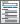Home  /  Products  /  Features  /  Finite mixture models (FMMs)

## Finite mixture models (FMMs)

• Mixtures of regression models
• Mixtures of distributions
• With two, three, four, or more latent classes (components)

Model class membership• Predictors of class membership
• Multinomial logistic model

Starting values

• EM algorithm
• Fixed or random starting values
• Select number of random draws

Inferences

• Class membership
• Posterior class membership
• Predicted means, probabilities, counts
• For each latent class
• Marginal with respect to latent classes
• Marginal with respect to posterior latent classes
• Survivor function
• Density function
• Distribution function

Postestimation selector

• View and run all postestimation features for your command
• Automatically updated as estimation commands are run

Factor variables

• Automatically create indicators based on categorical variables
• Form interactions among discrete and continuous variables
• Include polynomial terms
• Perform contrasts of categories/levels
Watch Introduction to Factor Variables in Stata tutorials

Marginal analysis

• Estimated marginal means
• Marginal and partial effects
• Average marginal and partial effects
• Adjusted predictions, means, and effects
• Works with multiple outcomes simultaneously
• Contrasts of margins
• Pairwise comparisons of margins
• Profile plots
• Graphs of margins and marginal effects